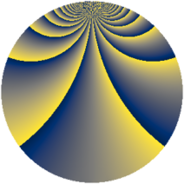# Properties

 Label 552.2.bbLevel $552$ Weight $2$ Character orbit 552.bb Rep. character $\chi_{552}(13,\cdot)$ Character field $\Q(\zeta_{22})$ Dimension $480$ Newform subspaces $1$ Sturm bound $192$ Trace bound $0$

# Related objects

## Defining parameters

 Level: $$N$$ $$=$$ $$552 = 2^{3} \cdot 3 \cdot 23$$ Weight: $$k$$ $$=$$ $$2$$ Character orbit: $$[\chi]$$ $$=$$ 552.bb (of order $$22$$ and degree $$10$$) Character conductor: $$\operatorname{cond}(\chi)$$ $$=$$ $$184$$ Character field: $$\Q(\zeta_{22})$$ Newform subspaces: $$1$$ Sturm bound: $$192$$ Trace bound: $$0$$

## Dimensions

The following table gives the dimensions of various subspaces of $$M_{2}(552, [\chi])$$.

Total New Old
Modular forms 1000 480 520
Cusp forms 920 480 440
Eisenstein series 80 0 80

## Trace form

 $$480q + 4q^{2} + 4q^{6} + 8q^{7} + 4q^{8} + 48q^{9} + O(q^{10})$$ $$480q + 4q^{2} + 4q^{6} + 8q^{7} + 4q^{8} + 48q^{9} - 4q^{10} - 4q^{14} - 8q^{15} - 8q^{16} - 4q^{18} + 20q^{20} + 20q^{22} - 8q^{23} - 4q^{24} + 48q^{25} + 16q^{30} + 16q^{31} + 4q^{32} + 6q^{34} - 22q^{36} + 90q^{38} - 74q^{40} + 90q^{42} - 130q^{44} + 96q^{46} - 88q^{48} - 48q^{49} + 142q^{50} - 142q^{52} + 18q^{54} - 82q^{56} + 22q^{58} + 2q^{60} - 40q^{62} - 8q^{63} + 16q^{66} - 44q^{68} + 16q^{71} - 4q^{72} + 10q^{74} - 138q^{76} - 40q^{79} - 170q^{80} - 48q^{81} - 124q^{82} - 8q^{84} - 216q^{86} - 108q^{88} + 4q^{90} - 198q^{92} - 238q^{94} + 80q^{95} + 4q^{96} - 132q^{98} + O(q^{100})$$

## Decomposition of $$S_{2}^{\mathrm{new}}(552, [\chi])$$ into newform subspaces

Label Dim. $$A$$ Field CM Traces $q$-expansion
$$a_2$$ $$a_3$$ $$a_5$$ $$a_7$$
552.2.bb.a $$480$$ $$4.408$$ None $$4$$ $$0$$ $$0$$ $$8$$

## Decomposition of $$S_{2}^{\mathrm{old}}(552, [\chi])$$ into lower level spaces

$$S_{2}^{\mathrm{old}}(552, [\chi]) \cong$$ $$S_{2}^{\mathrm{new}}(184, [\chi])$$$$^{\oplus 2}$$